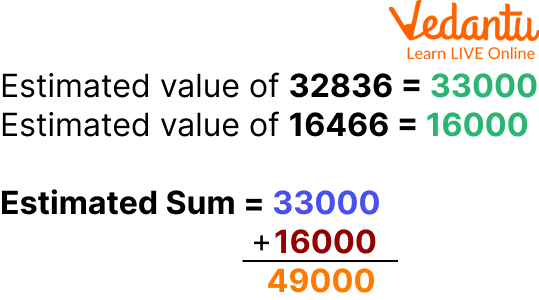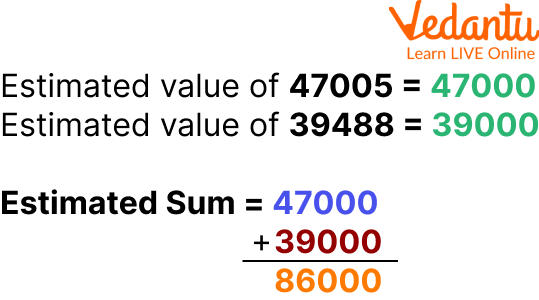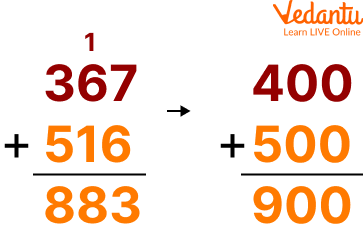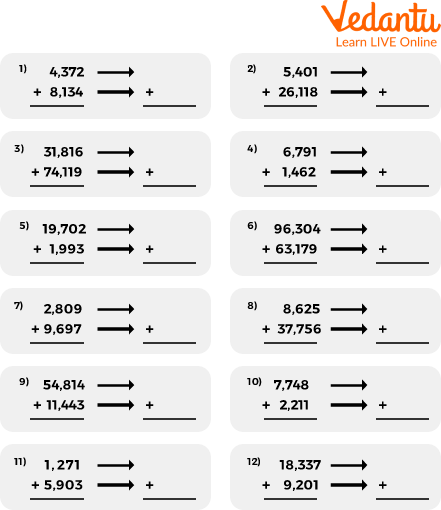Courses
Courses for Kids
Free study material
Offline Centres
More

# Estimate the Sum to the Nearest ThousandLast updated date: 01st Dec 2023
Total views: 98.4k
Views today: 2.98k## Introduction to Estimate Nearest Thousand

Estimating the sum to the nearest thousand is a common task that people often find hard. It requires a lot of mental math skills, and it's not easy to do in your head. Estimating the sum to the nearest thousand is a common math problem that people often get wrong in a lot of ways. The most common way people get it wrong is by rounding up or down when they should be estimating the number without any rounding.

The fundamentals of estimating the amount to the nearest thousand will be covered in this article. Writing down and adding up all of your numbers is the first step. Once you have done this, you can use a calculator to find out how many zeros are in that number. The number of zeros in your answer will tell you how many thousands there are in your answer.

## Estimation

The estimation of a number is the process of making a reasonable guess about the actual value in order to make calculations easier and more realistic. Estimation means approximating a quantity to the degree of accuracy required. To get a quick and rough answer, round off the numbers involved in the calculation.

Let's say you want to calculate the total cost of a few different products on your grocery list or you want to purchase a few gifts.

How would you estimate how much money you would need? Given that money is involved and calculations are made easier when prices are rounded to the closest tenth, you would do so. You might want to round a number to the next million or billion if you're dealing with exceptionally huge figures. And this is how place value is used in estimation.

## Estimate the Sum to the Nearest Thousand for Steps

Here are some steps related to estimating the sum to the nearest thousand, which is like this;

• First, we obtain a number that roughly approximates the total to the nearest thousand.

• Check the numbers in the hundreds place after that.

• The sum of the nearest thousand should be approximated if the digit in the hundreds place is greater than 5 or 5.

• Multiply the thousands digit by 1 and replace the hundreds, tens, and units digits with 0, keeping all other numbers the same.

• Approximate the sum of the nearest thousand if the digit in the hundreds place place is less than 5 for the corresponding number, then replace each digit with a 0 in the hundreds, tens and units or units place. Keep all other numbers as they are.

• In this way the sum of the nearest thousand is the number that is rounded off to the nearest thousand.

## Estimate the Sum to the Nearest Thousand for Table

Here are some table related to estimating the sum to the nearest thousand, which is like this:

 Number Nearest thousand Number 14729 15000 14329 14000 13578 14000 27803 28000 36529 37000 57842 58000 68945 69000

## Estimate the Sum to the Nearest Thousand Related Example

Here are some examples related to estimating the sum of the nearest thousand, which is like this;

Example: Estimate the sum by rounding off to nearest thousand for 16466 and 32836?

Ans: Estimate the sum of 16466 and 32836 to the nearest thousand.Example

Example: Estimate each sum to the nearest thousand for 39488 and 47005.

Ans: Estimate the sum of 39488 and 47005 to the nearest thousand.Example

Example: Estimate the sum of 367 and 516 to the nearest hundred?

Ans: Estimate the sum of 367 and 516 to the nearest hundred.Example

## Estimate the Sum to the Nearest Thousand Related Worksheet

Here are some worksheets related to estimating the sum of the nearest thousand, which is like this;Worksheet

## Summary

This is a very simple calculation that we use every day. It can be done by hand or with a calculator, but it is time-consuming and tedious. Estimating the sum to the nearest thousand is a very simple calculation that we use every day.

In the conclusion of this article, we will be discussing what we have learned and what we can do to make our estimation more accurate. In the first part of this article, I discussed why it is important for us to estimate a sum to the nearest thousand. In the second part, I discussed how you can estimate a sum using your knowledge of integers.

## FAQs on Estimate the Sum to the Nearest Thousand

1. What are the steps to calculate the sum to the nearest thousand in Maths?

This section will discuss the steps to calculate the sum to the nearest thousand in maths.

There are a few steps to calculate the sum to the nearest thousand in maths.

• First, find the difference between two numbers and subtract it from one number.

• Second, take that number and divide it by 10 and multiply it by 100.

• Third, take that number minus 100 and multiply it by 10.

2. What is the sum to the nearest thousand in Maths?

The sum to the nearest thousand is the sum of a number to the nearest thousand. It is a way to find out how many hundreds, tens, or ones are in a number.

3. What is the definition of "sum to the nearest thousand in Maths"?

In mathematics, the number "1,000" is called a "thousand." It is equal to one-thousand units. It is also equal to one-hundred multiplied by ten. The definition of "sum to the nearest thousand in maths" is a calculation that determines the sum of all numbers up to 1,000.